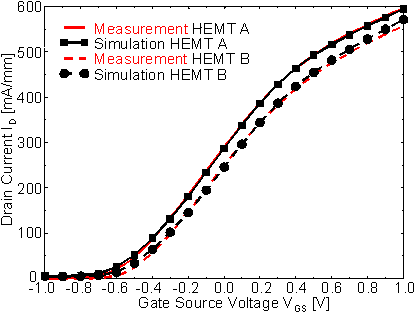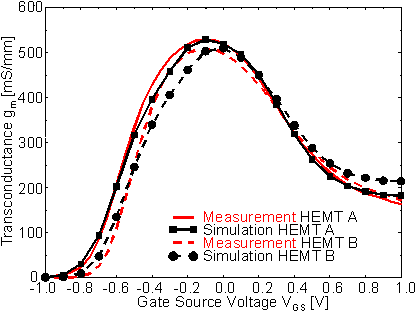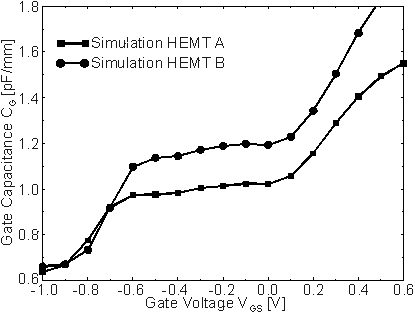Next: 6.1.1.2 Comparison of HEMT A and HEMT C Up: 6.1.1 DC Characteristics Previous: 6.1.1 DC Characteristics

6.1.1.1 Comparison of HEMT A and HEMT B

Measured and simulated transfer characteristics of HEMTs A and B are shown in  Figure 6.7. Although the parameter fit procedure described above was only applied to HEMT A, also HEMT B is simulated precisely. Both measurements and simulations show that the drain current of HEMT A (LG = 170 nm) is larger than the current of HEMT B (LG = 240 nm) by about 40 mA/mm. As can be seen in  Figure 6.8, also the maximum transconductance of HEMT A is superior to that of HEMT B by approximately 20 mS/mm, again measured as well as simulated. These results reflect the faster carrier transport due to the shorter gate of HEMT A.Figure 6.7 Measured and simulated transfer characteristics of HEMT A and HEMT B at VDS = 2.0 VFigure 6.8 Measured and simulated transconductance of HEMT A and HEMT B at VDS = 2.0 V

If the larger gm max of HEMT A were not caused by accelerated transport but by a distance dGC smaller than estimated in  Table 6.1, one would expect it to be correlated with a smaller ID instead of a larger one. This comparison with experiment provides evidence that the simulation is able to model gm ext and ID of both HEMTs in a consistent and realistic manner.

However, there is also one small detail shown in  Figure 6.8 in which simulation and measurement do not agree completely: the gate-source voltage VGS for which gm max is measured for HEMT B is slightly more negative than the simulated one. The reason for this behavior is not clear. As the two HEMTs were not fabricated in the same lot, small differences in the semiconductor passivation interface states could occur.

On the other hand, the experimental observation that the transconductance of HEMT A decreases more rapidly with increasing positive VGS than that of HEMT B is accurately reproduced by the simulation. As described in Chapter 5 the physical origin of this effect is the stronger real space transfer of electrons from the channel into the low-mobility barrier layer in the device with the shorter gate (HEMT A). The increase of real space transfer in short channel devices is also leading to a higher output conductance. This will be discussed in Section 6.1.2.
Figure 6.9 shows the simulated gate capacitances CG of HEMTs A and B. For VGS below pinchoff (i. e. VGS < -0.9 V), CG consists of the gate-drain capacitance CGD and parasitic contributions including the fringe capacitances. These values are very similar for both transistors. When VGS increases, the longer gate of HEMT B manifests itself in a stronger increase DCG compared to HEMT A. For HEMT B, DCGB » 520 fF, and for HEMT A, DCGA » 350 fF. The ratio DCGB /DCGA » 520/350 » 1.48 is close to the ratio of the gate lengths LGB/LGA » 240/170 » 1.41, as expected.Figure 6.9 Simulated CG of HEMT A and HEMT B at VDS = 2.0 VNext: 6.1.1.2 Comparison of HEMT A and HEMT C Up: 6.1.1 DC Characteristics Previous: 6.1.1 DC Characteristics

Helmut Brech
1998-03-11